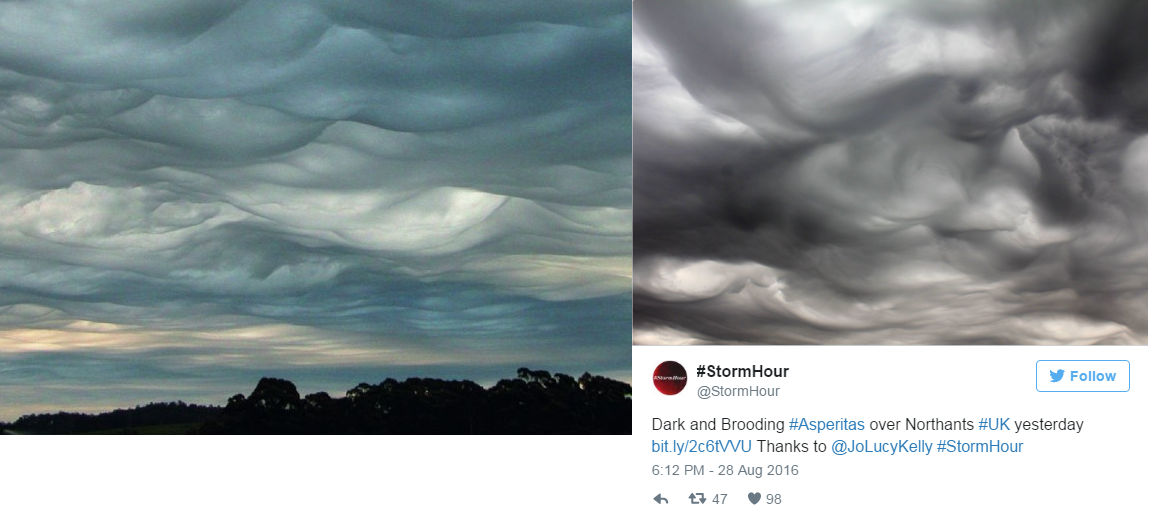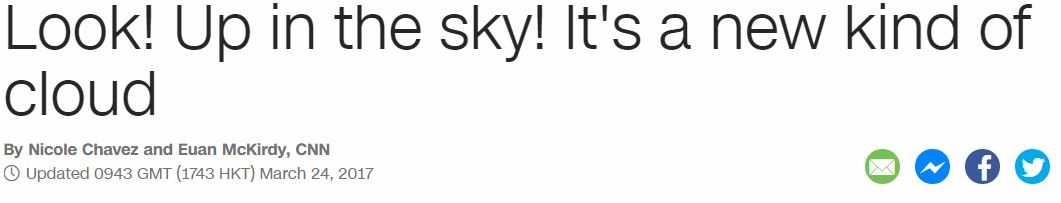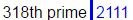## Cipher## Mar 24, 2017

### CNN - New kind of cloud - Are we going to start seeing more of these?

Something tells me that we are gonna start seeing more of these clouds being produced in the future...  they are surely not stopping with weather modification any time soon..

These are some of the pictures from their postLook! Up in the sky! Its a new kind of cloud = 30+60+60+20 + 300+70 + 9+50 + 200+8+5 + 100+20+700 + 9+200+100 + 1 + 50+5+500 + 20+9+50+4 + 60+6 + 3+30+60+300+4 = 3043 (English)
7x7x7 = 343 (227 is 343 in octal numbering system)
The story is released on "March Twenty fourth" = 30+1+80+3+8 + 100+900+5+40+100+400 + 6+50+200+80+100+8 = 2111 (Jewish)7/22 = 0.318
22/7 = 3.14

"The World Meteorological Organization had not updated the atlas in 30 years, until now."

Astrologers usually round out Saturn's orbit at 30 years, lately I've started noticing more and more how they love to do things 30 years apart
three decades = 7+19+9+22+22 + 23+22+24+26+23+22+8 = 227 (Reverse Ordinal)
"The cube of Saturn" = 7+19+22 + 24+6+25+22 + 12+21 + 8+26+7+6+9+13 = 227 (Reverse Ordinal)They are calling it "Asperitas cloud" = 1+1+7+5+9+9+2+1+1 + 3+3+6+3+4 = 55 (Reduced)
"cloud" = 3+12+15+21+4 = 55 (Ordinal)
"sky" = 19+11+25 = 55 (Ordinal)
"heaven" = 8+5+1+22+5+14 = 55 (Ordinal)

Note that World Meteorological day comes on 3/23, a day after 3/22, the number of Skull & Bones
The cloud was officially recognized on 3/23 but CNN is reporting it later for coding numerological reasons
"March Twenty Third" = 14+26+9+24+19 + 7+4+22+13+7+2 + 7+19+18+9+23 = 223 (Reverse Ordinal)
"Asperitas clouds" = 26+8+11+22+9+18+7+26+8 + 24+15+12+6+23+8 = 223 (Reverse Ordinal)
"Two two three" = 2+5+6 + 2+5+6 + 2+8+9+5+5 = 55 (Reduced)